Function Repository Resource:

# MoistAirRefractiveIndex

Compute the refractive index of moist air

Contributed by: Julián Laverde
 ResourceFunction["MoistAirRefractiveIndex"][assoc] returns the refractive index of moist air for the condition specified in the association assoc.

## Details

ResourceFunction["MoistAirRefractiveIndex"] returns the refractive index of moist air, corresponding to the selected temperature and pressure at the given wavelength.
The refractive index is the ratio between the speed of light in medium to speed in a vacuum.
Available elements in assoc and their default values are shown below:
 "Wavelength" 0.6 μm incident light wavelength "Temperature" 23°C temperature of the moist air "Pressure" 1 bar pressure of the moist air "RelativeHumidity" 50% relative humidity of the moist air "CarbonDioxideLevel" 370 ppm CO2 level of the moist air
ResourceFunction["MoistAirRefractiveIndex"][] gives a value for the standard conditions shown as defaults above.
The value for "Wavelength" can be any quantity whose physical dimension is "LengthUnit". If given only as a number, the default unit of wavelength is micrometers. The valid ranges of wavelength are from 0.3 μm to 5.2 μm, from 7.5 μm to 14.1 μm and from 16 μm to 24 μm.
The value for "Temperature" can be any quantity whose physical dimension is "TemperatureUnit". If given only as a number, the default unit of temperature is degrees Celsius.
The value of "Pressure" can be any quantity whose physical dimension is "PressureUnit". If given only as a number, the default unit of pressure is bars.
The value of "RelativeHumidity" can be any quantity whose unit is Percent. If given only as a number, the default unit of relative humidity is Percent.
There are two available ranges for "Pressure", "RelativeHumidity" and "Temperature" depending on the wavelength of the incident light.
The available ranges for 0.3 μm wavelength < 1.3 μm are:
 "Temperature" from -60°C to 100°C "Pressure" from 0.8 bars to 1.2 bars "RelativeHumidity" from 0% to 100%
The available ranges for 1.3 μm wavelength 5.2 μm, 7.5 μm wavelength 14.1 μm and 16 μm wavelength 24 μm are:
 "Temperature" from 10°C to 26.85°C "Pressure" from 0.5 bars to 1.223 bars "RelativeHumidity" from 5% to 60%
The value of "CarbonDioxideLevel" can be any parts-per notation quantity. If given only as a number, the default unit of carbon dioxide level is "PartsPerMillion". The valid range of carbon dioxide level is from 100 ppm to 500ppm.

## Examples

### Basic Examples (2)

Compute a refractive index for standard conditions:

 In:=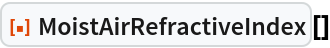Out=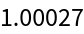Find the refractive index for a specified wavelength, temperature, pressure, relative humidity and carbon dioxide level:

 In:=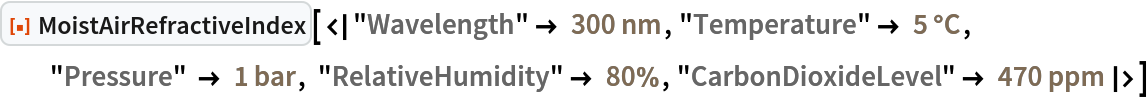Out=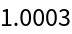### Scope (1)

If the units of the input are not specified, it takes the default units:

 In:=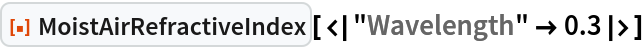Out=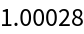### Properties and Relations (1)

Use Quantity to specify the units of the inputs:

 In:=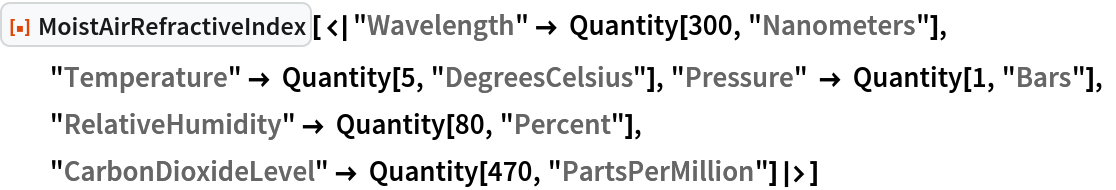Out=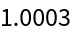### Possible Issues (1)

The units of the inputs must be valid length, temperature, pressure, percent or parts-per units:

 In:=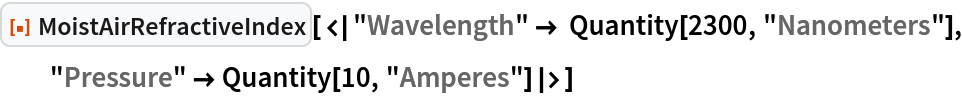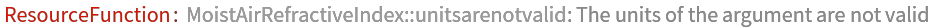Out=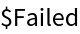### Neat Examples (1)

The function can be supplied to any suitable visualization function:

 In:=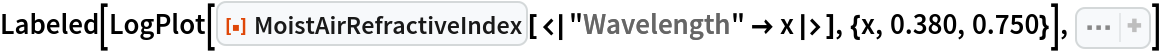Out=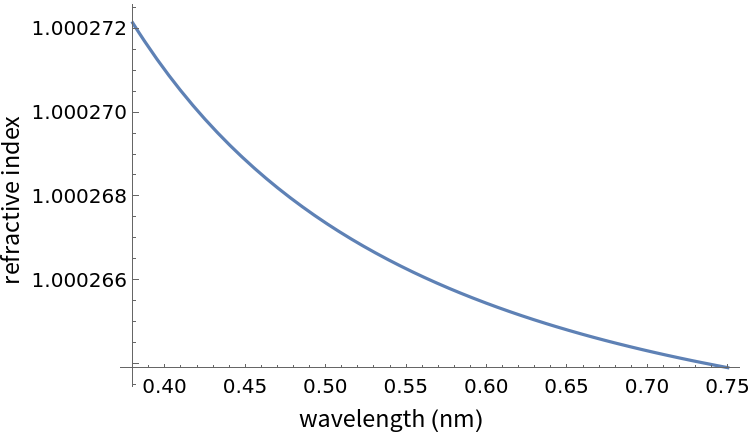## Publisher

WolframSpecialProjects

## Version History

• 1.0.0 – 08 January 2021